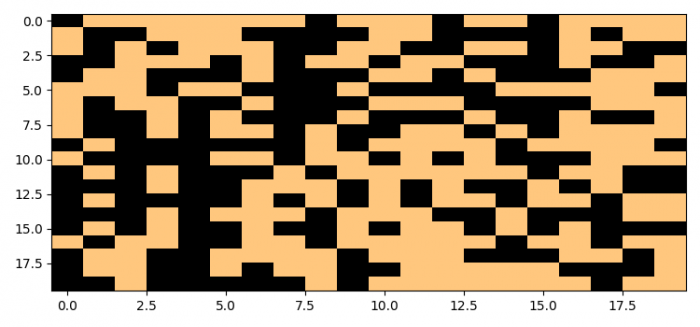# How to plot true/false or active/deactive data in Matplotlib?

MatplotlibPythonData Visualization

#### Python Data Science basics with Numpy, Pandas and Matplotlib

Most Popular

63 Lectures 6 hours

#### Data Visualization using MatPlotLib & Seaborn

11 Lectures 4 hours

#### MatPlotLib with Python

9 Lectures 2.5 hours

To plot true/false or active/deactive data in Matplotlib, we can take the following steps −

• Set the figure size and adjust the padding between and around the subplots.
• Create data using numpy with True or False.
• Create a new figure or activate an existing figure using figure() method.
• Add an '~.axes.Axes' to the figure as part of a subplot arrangement.
• Use imshow() method to display data as an image, i.e., on a 2D regular raster.
• To display the figure, use show() method.

## Example

import matplotlib.pyplot as plt
import numpy as np

plt.rcParams["figure.figsize"] = [7.50, 3.50]
plt.rcParams["figure.autolayout"] = True

data = np.random.random((20, 20)) > 0.5
fig = plt.figure()
plt.show()